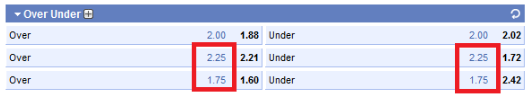# Asian total

For your better understanding of the notion of Asian total you should read the article about Betting Totals first. There were considered general ideas along with specific examples for those who’s about to bet.

Asian total consists of two total wagers divided by 0.5. All bets like TO 2.75, TO 2.25, TU 2.75, TU 2.25 are Asian totals. As you have noticed, it is marked as a usual total. The difference is in the meaning. Thus, Asian total bet TO 2.75 represents two wagers - TO 2.5 and TO 3 (2.5 and 3 divided by 0.5). In fact, TO 2.25 equals TO 2 and TO 2.5. The same with TU wagers. In that case, you certainly would ask about the way the bets are assessed: they are equally divided into two.

Let’s view some examples: winning, losing ones and those that might be returned. These bets are the most widespread in football. Here we may see Athletic Bilbao B vs Real Zaragoza

(Spain Liga Adelante) with two different bookies. The first one is Sbobet sportsbook:Asian total marked red.

188bet sportsbook offers it a bit differently:Similarly to the previous example, Asian total over 2/2.5 (marked blue) will equal to TO 2.25, and Under 1.5/2 - TU 1.75 (marked violet).

And now, let’s calculate the wager (188Bet): Over 2/2.5 (marked blue).
Let us suppose that Athletic Bilbao B vs Real Zaragoza ended the game 2-1. Score total is 2+1=3. Divide Asian total into parts: TO 2 and TO 2.5. Your bet will succeed (3>2 and 3>2.5).

In case the score is 2-0 or 1-1, the bookie returns your half bet on Over 2/2.5. Score total is 2. Consequently, the part of TO 2.5 will lose as 2<2.5. The other part of TO 2 will be calculated with 1 (as 2=2). Thus, you place \$10 on Over 2/2.5 and bookie gives you back \$5.

And the last variant. Team score <2. Bet on TO 2/2.5 will lose.

We can draw some conclusions that depending on the outcome you either win, lose or your stake may be half returned.

Rating:
5.0 from 5 based on 1 votes.